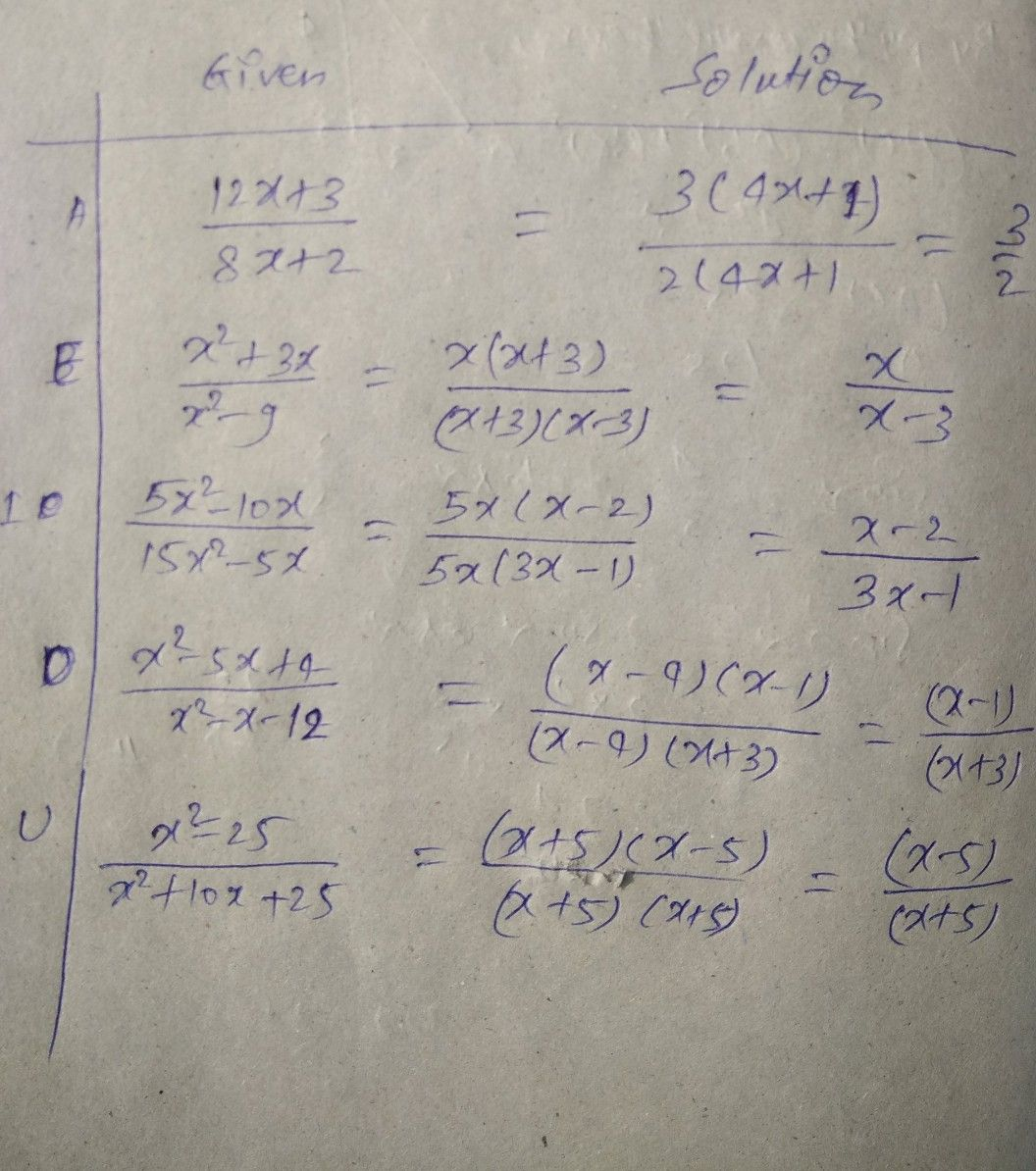Symbol
Problem$G$ Given Solution $A$ $\dfrac {12x+3} {8x+2}$ $E$ $\dfrac {x^{2}+3x} {x^{2-9}}$ $1$ $\dfrac {5x^{2}-10x} {15x2-5x}$ $0$ $\dfrac {x^{2}-5x+4} {x^{2-x-12}}$ $0$ $\dfrac {x^{2}-25} {x2+10x+25}$